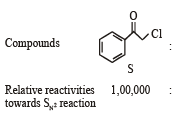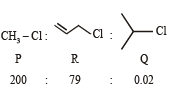Courses

# Test: Single Correct MCQs: Organic Chemistry - Some Basic Principles & Technique | JEE Advanced

## 56 Questions MCQ Test Chemistry 35 Years JEE Main & Advanced Past year Papers | Test: Single Correct MCQs: Organic Chemistry - Some Basic Principles & Technique | JEE Advanced

Description
This mock test of Test: Single Correct MCQs: Organic Chemistry - Some Basic Principles & Technique | JEE Advanced for JEE helps you for every JEE entrance exam. This contains 56 Multiple Choice Questions for JEE Test: Single Correct MCQs: Organic Chemistry - Some Basic Principles & Technique | JEE Advanced (mcq) to study with solutions a complete question bank. The solved questions answers in this Test: Single Correct MCQs: Organic Chemistry - Some Basic Principles & Technique | JEE Advanced quiz give you a good mix of easy questions and tough questions. JEE students definitely take this Test: Single Correct MCQs: Organic Chemistry - Some Basic Principles & Technique | JEE Advanced exercise for a better result in the exam. You can find other Test: Single Correct MCQs: Organic Chemistry - Some Basic Principles & Technique | JEE Advanced extra questions, long questions & short questions for JEE on EduRev as well by searching above.
QUESTION: 1

### The bond order of in dividual carbon -carbon bonds in benzene is

Solution:

NOTE : The phenomenon of resonance gives identical bonding and hence identical bond lengths.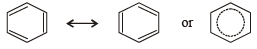C – C bond order in benzene = 1.5

QUESTION: 2

### Molecule in which the distance between the two adjacent carbon atoms is largest is

Solution:

TIPS/Formulae :

The bond length decreases in the order.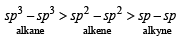On the basis of the size of the hybrid orbitals, sp orbital should form the shortest and sp3 orbital the longest bond with other atom.

QUESTION: 3

### The compound which is not isomeric with diethyl ether is

Solution:

The first three are isomers of diethyl ether, C2H5OC2H5 (C4H10O).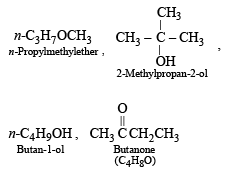QUESTION: 4

Among the following, the compound that can be most readily sulphonated is

Solution:

TIPS/FORMULAE :

–NO2, –Cl and –OH are electron-attracting or withdrawing group due to –M, –E and/or –I effects where as –CH3 show, +I effect (electron releasing).
Because of the + I effect of the CH3 group, toluene has the highest electron density in the o- and p- positions and hence can be most readily sulphonated.

QUESTION: 5

Solution: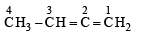Hybridisation in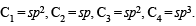QUESTION: 6

Which of the following compounds will exhibit cis-trans (geometrical) isomerism?

Solution:

TIPS/Formulae :
cis-trans – Isomerism is due to restricted rotation either due to carbon-carbon double bond or due to cyclic structure.
NOTE : Geometrical Isomerism : The isomers which possess the same structural formula but differ in the spatial arrangement of the groups around the double bond are known as geometrical isomers.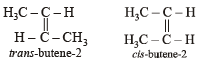QUESTION: 7

The IUPAC name of the compound having the formula is: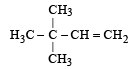Solution: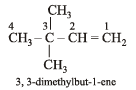QUESTION: 8

An isomer of ethanol is :

Solution: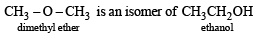QUESTION: 9

Out of the following compounds, which will have a zero dipole moment?

Solution: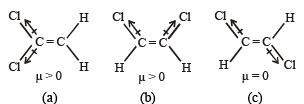[Note : dipole moment is a vector quantity].

QUESTION: 10

The bond between carbon atom (1) and carbon atom (2) in compound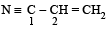involves the hybrids as

Solution:

Car bon bonded with a triple bond (i.e. C1) is sp hybridised. Carbon bonded with a double bond (C2) is sp2 hybridised.

QUESTION: 11

The IUPAC name of the compound

CH2 = CH – CH (CH3)2 is

Solution: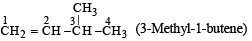QUESTION: 12

The number of isomers of C6H14 is

Solution:

TIPS/Formulae :
For knowing the possible isomers of the compound follow the following points.
(i) First write down the possible number of isomeric parent alkane.
(ii) Introduce the given functional group at different positions so as to get different isomeric compound.
There are 5 isomers possible for C6H14.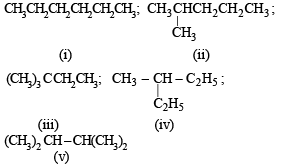QUESTION: 13

The Cl—C—Cl angle in 1,1,2,2-tetrachloroethene and tetrachloromethane respectively will be about

Solution:

The bond angle in sp3, sp2 and sp hybridization is respectively 109.28', 120º and 180º.
Tetrachloroethene being an alkene has sp2 hybridised C-atoms and hence the Cl – C –  Cl angle is 120º, whereas in tetrachloromethane, carbon is sp3 hybridised, so the angle is 109º.28’.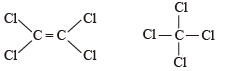QUESTION: 14

The compound which has one isopropyl group is :

Solution: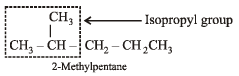QUESTION: 15

The C–H bond distance is the longest in :

Solution:

C2H6 is a saturated hydrocarbon and its carbon is sp3 hybridised. Hence it is least electronegative among alkanes, alkenes and akynes with the result C – H bond length will be maximum.

QUESTION: 16

The number of sigma and pi-bonds in 1-butene-3-yne are :

Solution:

CH2 = CH – C ≡ CH;
No of s bonds = 2 + 1 + 1 + 1 + 1 +1 = 7;
No of  π bonds = 1 + 2 = 3

QUESTION: 17

In CH3CH2OH, the bond that undergoes heterolytic cleavage most readily is

Solution:

NOTE : Heterolytic fission occurs when the two atoms differ considerably in their electronegativities.
O – H bond undergoes cleavage most readily because O and H differ markedly in their electronegativity and further oxygen being highly electronegative can accommodate the negative charge more effectively developed after the cleavage.

QUESTION: 18

The compound which gives the most stable carbonium ion on dehydration is :

Solution:

NOTE : The order of stability of carbonium ion is

tertiary > secondary > primary > methyl

Tertiary carbonium ions (formed in b) are more stable because of electron repelling (+I effect) nature of CH3 group due to which the +ve charge gets dispersed and also due to hyperconjugation.

QUESTION: 19

The hybridization of carbon atoms in C–C single bond of HC ≡ C – CH = CH2 is

Solution: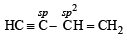QUESTION: 20

The products of combustion of an aliphatic thiol (RSH) at 298 K are

Solution: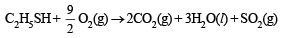At 298 K, CO2 and SO2 exist as gases while H2O exists as liquid.

QUESTION: 21

Isomers which can be inter converted th rough rotation around a single bond are

Solution:

Stereoisomers which are mirror image of each other are enantiomers and the one which are not mirror images are diasteromers. Conformation of the molecule is the spatial arrangement of the atoms of a given molecular structure that are obtained merely by rotation about a sigma bond in the molecule.

QUESTION: 22

The structure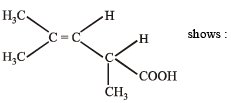Solution:

A compound which consists of at least one asymmetric carbon atom is capable of showing the phenomenon of optical isomerism.
The structure cannot show geometrical isomerism as one of the carbons along the double bond has identical group (methyl). Tautomerism is not possible because of the absence of –CO, group. It shows optical isomerism because it has chiral C atom with four different groups, H, CH3, COOH and (CH3),C = CH.

QUESTION: 23

Allyl isocyanide has :

Solution:The above structure of allyl isocyanide clearly shows 5 C – H (σ), 2 C – C (σ), 1C – N (σ), 1N – C(σ), 1C – C (π), 2N– C (π) bonds, i.e., 9σ and 3π bonds in all. There are 2 non-bonded electrons on the C-atom (co-ordinate bond between N and C, the electron pair of N is shifted towards C).

QUESTION: 24

Arrange in order of decreasing trend towards SE reactions :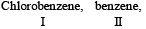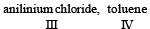Solution: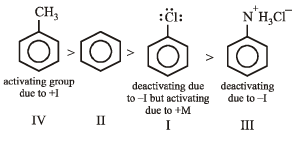QUESTION: 25

Most stable carbonium ion is :

Solution:

TIPS/Formulae :

The stability of carbonium ion is influenced by both resonance and inductive effect.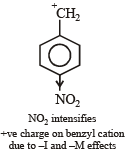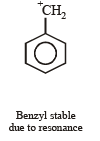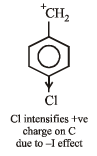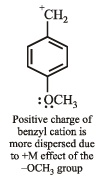QUESTION: 26

In the following compounds,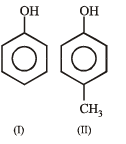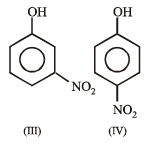The order of acidity is :

Solution:

NOTE : –NO2 is an electron-attracting group where as –CH3 is an electron-releasing group.

An electron - attracting substituent tends to dispersethe negative charge of the phenoxide ion and thus, makes it more stable. This, in turn, increases the acid strength of phenol. The substituent in para position is more effective than in the meta position as the former involves a resonating structure bearing negative charge on the carbon attached to the electron - withdrawing substituent.

An electron - releasing substituent tends to intensify the negative charge of the phenoxide ion and thus makes it more unstable. This, in turn, decreases the acid strength of phenol. Hence, the order of acid strength is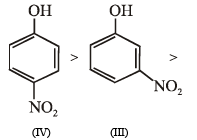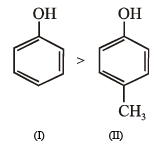QUESTION: 27

Arrange the following compounds in order of increasing dipole moment.

Toluene (I)
m-dichlorobenzene (II)
o-dichlorobenzene (III)
p-dichlorobenzene (IV)

Solution:

Dipole moment of p-dichlorobenzene is zero because of symmetrical structure. o- and m-dichlorobenzene have higher dipole moments than toluene due to high electronegativity of chlorine than –CH3 group. Further, the o-dichlorobenzene has higher dipole moment due to lower bond angle than the m-isomer. Hence, the order of increasing dipole moment is :
p-dichlorobenzene (IV)  <  toluene (I) < m-dichlorobenzene (II)  <  o-dichlorobenzene (III)

QUESTION: 28

How many optically active stereoisomers are possible for butane-2, 3-diol?

Solution:

The stereoisomers of butane -2,3-diol are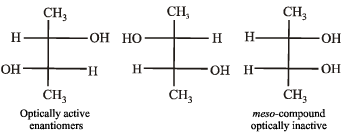QUESTION: 29

In the compound CH2 = CH–CH2–CH2–C ≡ CH, the C2–C3 bond is of the type,

Solution: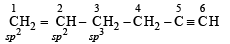QUESTION: 30

The optically active tartaric acid is named as D – (+) – tartaric acid because it has a positive

Solution:

TIPS/Formulae :

Glyceraldehyde is taken as arbitrary standard for D, L – nomenclature.

QUESTION: 31

Which of the following compounds will exhibit geometrical isomerism ?

Solution:

PhCH2CH= CHCH3 will exhibit geometrical isomerism because in others one of the doubly bonded carbon atom has two similar groups.

QUESTION: 32

Which of the following has the highest nucleophilicity?

Solution:

–CH3 is the best nucleophile because carbon is least electronegative among the given options. The order is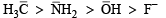QUESTION: 33

The order of reactivities of the following alkyl halides for a SN2 reaction is

Solution:

Rate of reaction will be R – I > R – Br > R – Cl > R – F. because I– is the best, while F– is the poorest leaving groups among halide ions.

QUESTION: 34

Which of the following has the most acidic hydrogen ?

Solution: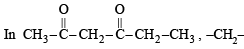group is flanked on both sides by electron- withdrawing groups and hence its hydrogens are most acidic. Once a carbanion is formed, it is stabilised due to resonance.

QUESTION: 35

The number of isomers for the compound with molecular formula C2BrClFI is

Solution:

Number of isomers (six) can be derived by keeping the position of any one halogen (say Br) fixed and changing the position of the other halogen one by one.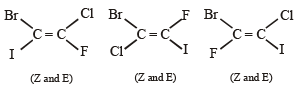QUESTION: 36

An SN2 reaction at an asymmetric carbon of a compound always gives

Solution:

SN2 reactions proceed with inversion of configuration.
Since the attacking nucleophile is not necessarily the same as that of leaving group, the product cannot be enantiomer of the substrate and thus necessarily will not have opposite optical rotation. Moreover since only one product is obtained, we can not obtain diastereomers.

QUESTION: 37

Which of the following compounds exhibits stereoisomerism?

Solution:

2-Methylbutanoic acid contains one asymmetric centre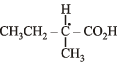QUESTION: 38

Which of the following acids has the smallest dissociation constant ?

Solution:

TIPS/Formulae :

(i) The inductive effect decreases with increase in distance of halogen atom from the carboxylic group and hence the strength of acid proportionally decreases.
(ii) Th e acidity in cr eas es with th e in cr eas e in electronegativity of the halogen present.
Smallest dissociation constant means weakest acid, which is BrCH2 CH2COOH because here Br (less electronegative than F) is two carbon atoms away from – COOH.

QUESTION: 39

Identify the correct order of boiling points of the following compounds;

CH3CH2CH2CH2OH, CH3CH2CH2CHO, CH3CH2CH2COOH

Solution:

In car boxylic acids, molecules ar e more str ongly associated followed by alcohols.

QUESTION: 40

Identify the correct order of reactivity in electrophilic substitution reactions of the following compounds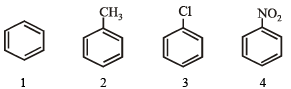Solution: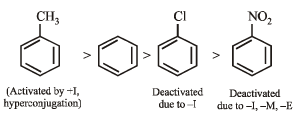QUESTION: 41

Which of the following hydrocarbons has the lowest dipole moment ?

Solution:

CH3C ≡ CCH3 is linear and symmetrical ; thus it has lowest dipole moment.

QUESTION: 42

Which of the following represents the given mode of hybridisation sp2 – sp2 – sp – sp from left to right?

Solution:

A has the following hybridization order: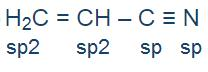Nitrogen atom has an sp hybridization because it has three bond pairs and one lone pair.

Note: lone pair and negative charge on an atom can be converted to a bond while considering the hybridization.

B has the following hybridization order: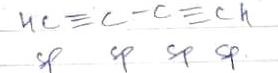C has the following hybridization order: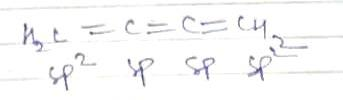D has the following hybridization order: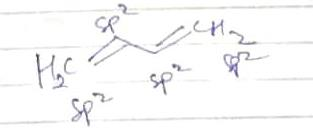Hence A is the correct answer.

QUESTION: 43

Among the following, the molecule with the highest dipole moment is:

Solution:

NOTE : Dipole moment is a vector quantity.
Methane molecule being symmetrical, has zero dipole moment. Replacement of one of the H– atoms by Cl atom increases the dipole moment. The increase in dipole moment is rather more than what can be expected because of the fact that the bond dipole moment of C – H bond and that of C – Cl bond reinforce one another.
Replacement of another H atom by Cl increases the bond angle due to lone pair – lone pair repulsion between two Cl–atoms thereby reducing the dipole moment of the molecule.  Increase in angle is again caused by the the introduction of the third Cl–atom.
When the fourth Cl–atom is introduced, the molecule (CCl4) again becomes symmetrical and dipole moment reduces to zero. So, CH3Cl will have the maximum dipole moment.

QUESTION: 44

In the given conformation, if C2 is rotated about C2 – C3 bond anticlockwise by an angle of 120º then the conformation obtained is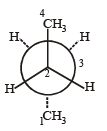Solution:

TIPS/Formulae : Any conformation between two extreme positions i.e. eclipsed and staggered is known as Gauche or Skew form.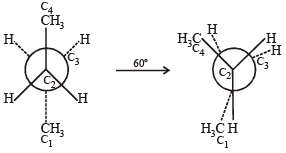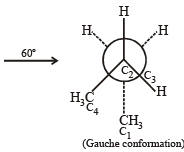QUESTION: 45

Which of the following resonating structures of 1-methoxy1,3-butadiene is least stable?

Solution:

Structures (a) and (b) are quite stable because here every atom has complete octet; in structures (c) and (d), every atom does not have complete octet; hence these are less stable than (a) and (b). However, structure (d) is stabilised by resonance, which is not possible in (c). Hence (c) is least stable.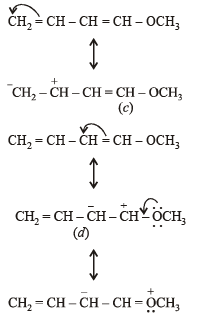QUESTION: 46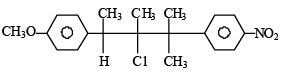compound on hydrolysis in aqueous acetone will give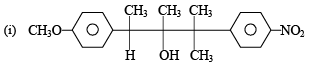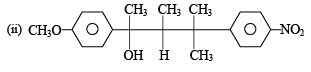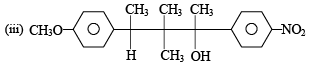Solution:

NOTE : This is an example of SN1 reaction involving carbocation as  intermediate.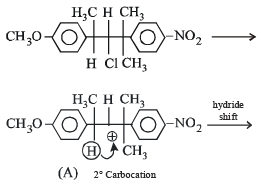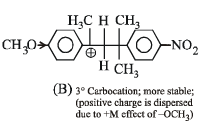This carbocation is especially stabilised through resonance in which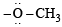group acts as a good electron donor.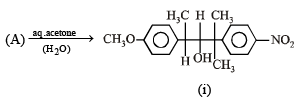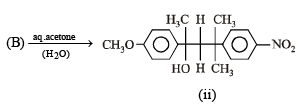QUESTION: 47

The IUPAC name of C6H5COCl is

Solution:

Carboxylic acids ar e named as oyl chlorides

QUESTION: 48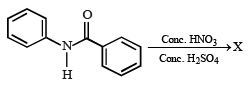the structure of the major product 'X' is

Solution:

The ring to which –NH group is attached is activated due to the lone pairs on N (+M and +E effects); while the ring to which –C = O is attached is deactivated.
Hene, the electrophile would go to the para-position of the activated ring.

QUESTION: 49

Among the following, the least stable resonance structure is

Solution:

Due to similar charges on adjacent atoms, the structure (a) is least stable.

QUESTION: 50

The number of stereoisomers obtained by bromination of trans-2-butene is

Solution:

Anti addition of Br2 on trans alkene provides meso compound.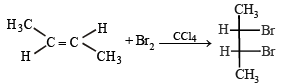QUESTION: 51

Hyperconjugation involves overlap of the following orbitals

Solution:

Alkyl groups with at least one hydr ogen atom on the a-carbon atom, attached to an unsaturated carbon atom, are able to release electrons in the following way.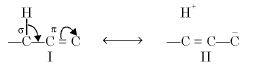Note that the delocalisation involves s and p bond orbitals (or p orbitals in case of free radicals) ; thus it is also known as σ – π conjugation. Th is type of electron release due to the presence of the system H—C—C = C is known as hyperconjugation

QUESTION: 52

The correct stability order for the following species is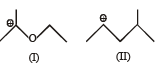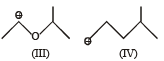Solution:

The correct stability order is

I    > III    > II  >   IV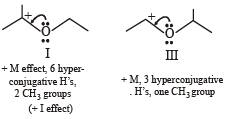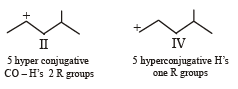QUESTION: 53

The IUPAC name of the following compound is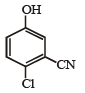Solution:

– CN has highest priority. Further the sum of locants is 7 in (b) and 9 in (d).

QUESTION: 54

In the following carbocation, H/CH3 that is most likely to migrate to the positively charged carbon is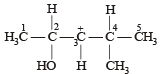Solution:

NOTE : Migrating tendency of hydride is greater than that of alkyl group. Further migration of hydride from C–2 gives more stable carbocation (stabilized by +R effect of OH group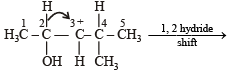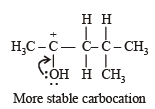QUESTION: 55

Among the following compounds, the most acidic is

Solution:

o-Hydroxyben zoic acid is str on gest acid an d th e decreasing order of acidity is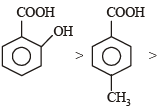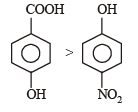QUESTION: 56

KI in acetone, undergoes SN2 reaction with each of P, Q, R and S. The rates of the reaction vary as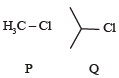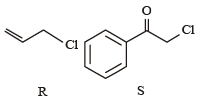Solution: